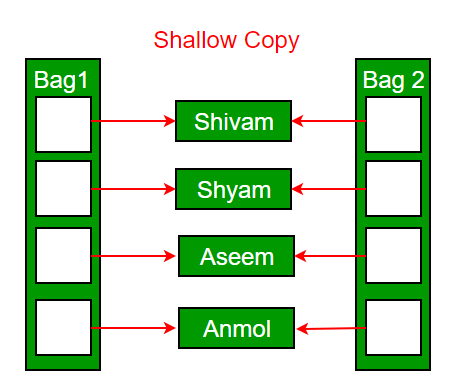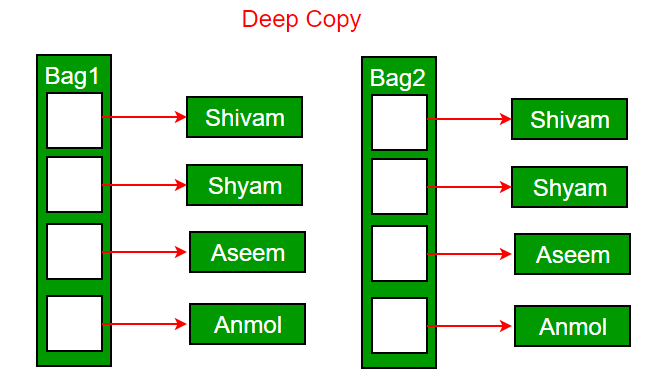Related Articles

# Copy Constructor in C++

• Difficulty Level : Hard
• Last Updated : 28 Jun, 2021

We have discussed an introduction to Constructors in C++. In this post, the copy constructor is discussed.
What is a copy constructor?
A copy constructor is a member function that initializes an object using another object of the same class. A copy constructor has the following general function prototype:

`    ClassName (const ClassName &old_obj); `

Following is a simple example of copy constructor.

## CPP

 `#include``using` `namespace` `std;` `class` `Point``{``private``:``    ``int` `x, y;``public``:``    ``Point(``int` `x1, ``int` `y1) { x = x1; y = y1; }` `    ``// Copy constructor``    ``Point(``const` `Point &p1) {x = p1.x; y = p1.y; }` `    ``int` `getX()            {  ``return` `x; }``    ``int` `getY()            {  ``return` `y; }``};` `int` `main()``{``    ``Point p1(10, 15); ``// Normal constructor is called here``    ``Point p2 = p1; ``// Copy constructor is called here` `    ``// Let us access values assigned by constructors``    ``cout << ``"p1.x = "` `<< p1.getX() << ``", p1.y = "` `<< p1.getY();``    ``cout << ``"\np2.x = "` `<< p2.getX() << ``", p2.y = "` `<< p2.getY();` `    ``return` `0;``}`

Output:

```p1.x = 10, p1.y = 15
p2.x = 10, p2.y = 15 ```

When is  copy constructor called?
In C++, a Copy Constructor may be called in the following cases:
1. When an object of the class is returned by value.
2. When an object of the class is passed (to a function) by value as an argument.
3. When an object is constructed based on another object of the same class.
4. When the compiler generates a temporary object.
It is, however, not guaranteed that a copy constructor will be called in all these cases, because the C++ Standard allows the compiler to optimize the copy away in certain cases, one example is the return value optimization (sometimes referred to as RVO)
Source: https://www.geeksforgeeks.org/g-fact-13/

When is a user-defined copy constructor needed?
If we don’t define our own copy constructor, the C++ compiler creates a default copy constructor for each class which does a member-wise copy between objects. The compiler created copy constructor works fine in general. We need to define our own copy constructor only if an object has pointers or any runtime allocation of the resource like filehandle, a network connection..etc.
The default constructor does only shallow copy.Deep copy is possible only with user defined copy constructor. In user defined copy constructor, we make sure that pointers (or references) of copied object point to new memory locations.Copy constructor vs Assignment Operator
Which of the following two statements call copy constructor and which one calls assignment operator?

## CPP

 `MyClass t1, t2;``MyClass t3 = t1;  ``// ----> (1)``t2 = t1;          ``// -----> (2)`

Copy constructor is called when a new object is created from an existing object, as a copy of the existing object. Assignment operator is called when an already initialized object is assigned a new value from another existing object. In the above example (1) calls copy constructor and (2) calls assignment operator. See this for more details.
Write an example class where copy constructor is needed?
Following is a complete C++ program to demonstrate use of Copy constructor. In the following String class, we must write copy constructor.

## CPP

 `#include``#include``using` `namespace` `std;` `class` `String``{``private``:``    ``char` `*s;``    ``int` `size;``public``:``    ``String(``const` `char` `*str = NULL); ``// constructor``    ``~String() { ``delete` `[] s;  }``// destructor``    ``String(``const` `String&); ``// copy constructor``    ``void` `print() { cout << s << endl; } ``// Function to print string``    ``void` `change(``const` `char` `*);  ``// Function to change``};` `String::String(``const` `char` `*str)``{``    ``size = ``strlen``(str);``    ``s = ``new` `char``[size+1];``    ``strcpy``(s, str);``}` `void` `String::change(``const` `char` `*str)``{``    ``delete` `[] s;``    ``size = ``strlen``(str);``    ``s = ``new` `char``[size+1];``    ``strcpy``(s, str);``}` `String::String(``const` `String& old_str)``{``    ``size = old_str.size;``    ``s = ``new` `char``[size+1];``    ``strcpy``(s, old_str.s);``}` `int` `main()``{``    ``String str1(``"GeeksQuiz"``);``    ``String str2 = str1;` `    ``str1.print(); ``// what is printed ?``    ``str2.print();` `    ``str2.change(``"GeeksforGeeks"``);` `    ``str1.print(); ``// what is printed now ?``    ``str2.print();``    ``return` `0;``}`

Output:

```GeeksQuiz
GeeksQuiz
GeeksQuiz
GeeksforGeeks```

What would be the problem if we remove copy constructor from above code?
If we remove copy constructor from the above program, we don’t get the expected output. The changes made to str2 reflect in str1 as well which is never expected.

## CPP

 `#include``#include``using` `namespace` `std;` `class` `String``{``private``:``    ``char` `*s;``    ``int` `size;``public``:``    ``String(``const` `char` `*str = NULL); ``// constructor``    ``~String() { ``delete` `[] s;  }``// destructor``    ``void` `print() { cout << s << endl; }``    ``void` `change(``const` `char` `*);  ``// Function to change``};` `String::String(``const` `char` `*str)``{``    ``size = ``strlen``(str);``    ``s = ``new` `char``[size+1];``    ``strcpy``(s, str);``}` `void` `String::change(``const` `char` `*str)``{``    ``delete` `[] s;``    ``size = ``strlen``(str);``    ``s = ``new` `char``[size+1];``    ``strcpy``(s, str);``}` `int` `main()``{``    ``String str1(``"GeeksQuiz"``);``    ``String str2 = str1;` `    ``str1.print(); ``// what is printed ?``    ``str2.print();` `    ``str2.change(``"GeeksforGeeks"``);` `    ``str1.print(); ``// what is printed now ?``    ``str2.print();``    ``return` `0;``}`

Output:

```GeeksQuiz
GeeksQuiz
GeeksforGeeks
GeeksforGeeks```

Can we make copy constructor private?
Yes, a copy constructor can be made private. When we make a copy constructor private in a class, objects of that class become non-copyable. This is particularly useful when our class has pointers or dynamically allocated resources. In such situations, we can either write our own copy constructor like above String example or make a private copy constructor so that users get compiler errors rather than surprises at runtime.
Why argument to a copy constructor must be passed as a reference?
A copy constructor is called when an object is passed by value. Copy constructor itself is a function. So if we pass an argument by value in a copy constructor, a call to copy constructor would be made to call copy constructor which becomes a non-terminating chain of calls. Therefore compiler doesn’t allow parameters to be passed by value.
Why argument to a copy constructor should be const?
See https://www.geeksforgeeks.org/copy-constructor-argument-const/